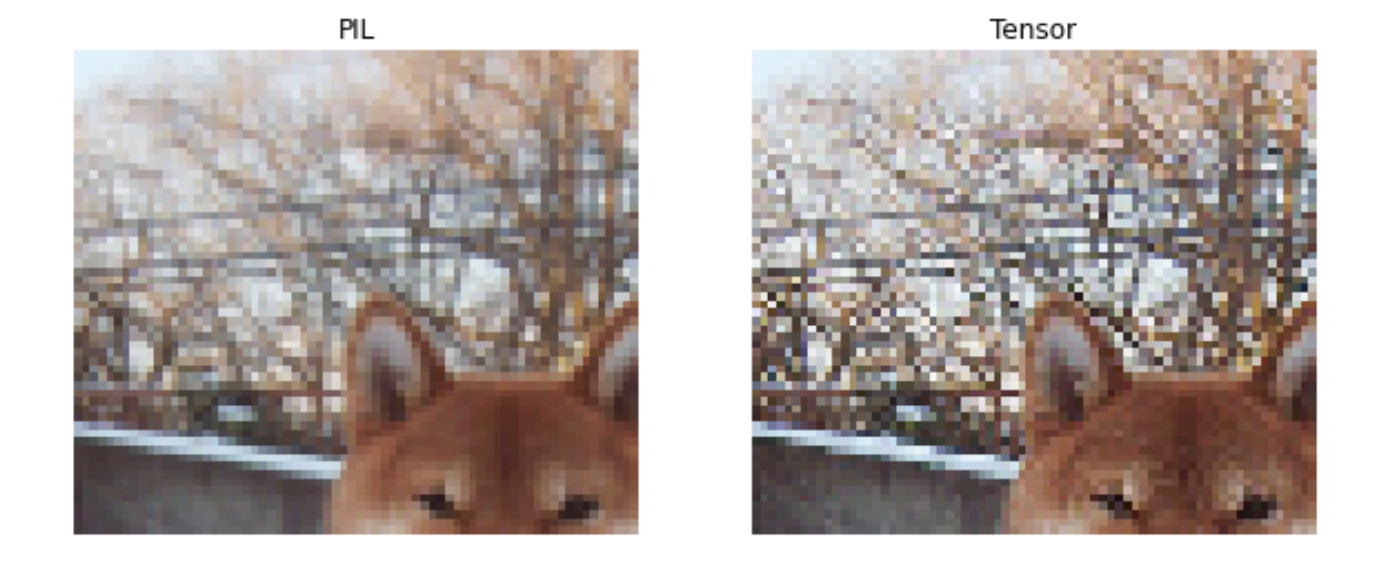Yesterday I was refactoring some code to put on our production code base. It is a simple image classifier trained with fastai. In our deployment env we are not including fastai as requirements and rely only on pure pytorch to process the data and make the inference. (I am waiting to finally be able to install only the fastai vision part, without the NLP dependencies, this is coming soon, probably in fastai 2.3, at least it is in Jeremy's roadmap). So, I have to make the reading and preprocessing of images as close as possible as fastai `Transform` pipeline, to get accurate model outputs.

After converting the transforms to `torchvision.transforms` I noticed that my model performance dropped significantly. Initially I thought that it was fastai's fault, but all the problem came from the new interaction between the `tochvision.io.images.read_image` and the `torchvision.transforms.Resize`. This transform can accept `PIL.Image.Image` or Tensors, in short, the resizing does not produce the same image, one is way softer than the other. The solution was not to use the new Tensor API and just use `PIL` as the image reader.

TL;DR :torchvision's `Resize` behaves differently if the input is a `PIL.Image` or a torch tensor from `read_image`. Be consistent at training / deploy.

Let's take a quick look on the preprocessing used for training and there corresponding torch version with the new tensor API as shown here

Below are the versions of fastai, fastcore, torch, and torchvision currently running at the time of writing this:

• `python` : 3.8.6
• `fastai` : 2.2.8
• `fastcore` : 1.3.19
• `torch` : 1.7.1
• `torch-cuda` : 11.0
• `torchvision` : 2.2.8: 0.8.2
Note: You can easily grab this info from `fastai.test_utils.show_install`

## A simple example

Let's make a simple classifier on the PETS dataset, for more details this comes from the fastai tutorial

let's grab the data

```path = untar_data(URLs.PETS)
files = get_image_files(path/"images")

def label_func(f):
return f.isupper()

dls = ImageDataLoaders.from_name_func(path, files, label_func, item_tfms=Resize((256, 192)))
```

A learner it is just a wrapper of Dataloaders and the model. We will grab an imagene pretrained `resnet18`, we don't really need to train it to illustrate the problem.

```learn = cnn_learner(dls, resnet18)
```

and grab one image (`load_image` comes from fastai and returns a memory loaded `PIL.Image.Image`)

```fname = files
img
``````learn.predict(fname)
```
`('False', tensor(0), tensor([0.7530, 0.2470]))`

Let's understand what is happening under the hood:

and we can call the prediction using fastai `predict` method, this will apply the same transforms as to the validation set.

• create PIL image
• Transform the image to pytorch Tensor
• Scale values by 255
• Normalize with imagenet stats

doing this by hand is extracting the preprocessing transforms:

```dls.valid.tfms
```
`(#2) [Pipeline: PILBase.create,Pipeline: partial -> Categorize -- {'vocab': None, 'sort': True, 'add_na': False}]`
```dls.valid.after_item
```
`Pipeline: Resize -- {'size': (192, 256), 'method': 'crop', 'pad_mode': 'reflection', 'resamples': (2, 0), 'p': 1.0} -> ToTensor`
```dls.valid.after_batch
```
`Pipeline: IntToFloatTensor -- {'div': 255.0, 'div_mask': 1}`

Let's put all transforms together on a fastcore `Pipeline`

```preprocess = Pipeline([Transform(PILImage.create),
Resize((256,192)),
ToTensor,
IntToFloatTensor,
Normalize.from_stats(*imagenet_stats)])
```

we can then preprocess the image:

```tfm_img = preprocess(fname)
tfm_img.shape
```
`torch.Size([1, 3, 256, 192])`

and we get the exact same predictions as before

```with torch.no_grad():
preds = learn.model(tfm_img).softmax(1)
preds
```
`tensor([[0.4149, 0.5851]])`

## Using torchvision preprocessing

Now let's try to replace fastai transforms with torchvision

```import PIL
import torchvision.transforms as T
```
```pil_image = load_image(fname)
pil_image
``````type(pil_image)
```
`PIL.Image.Image`

let's first resize the image, we can do this directly over the `PIL.Image.Image` or using `T.Resize` that works both on `IPIL` images or `Tensor`s

```resize = T.Resize([256, 192])
res_pil_image = resize(pil_image)
```

we can then use `T.ToTensor` this will actually scale by 255 and transform to tensor, it is equivalent to both `ToTensor` + `IntToFloatTensor` from fastai.

```timg = T.ToTensor()(res_pil_image)
```

then we have to normalize it:

```norm = T.Normalize(*imagenet_stats)
nimg = norm(timg).unsqueeze(0)
```

and we get almost and identical results! ouff.....

```with torch.no_grad():
preds = learn.model(nimg).softmax(1)
preds
```
`tensor([[0.4149, 0.5851]])`

## Torchvision new Tensor API

Let's try this new Tensor based API that torchvision introduced on `v0.8` then!

```import torchvision.transforms as T
```

`read_image` is pretty neat, it actually read directly the image to a pytorch tensor, so no need for external image libraries. Using this API has many advantages, as one can group the model and part of the preprocessing as whole, and then export to torchscript all together: model + preprocessing, as shown in the example here

```timg = read_image(str(fname)) # it is sad that it does not support pathlib objects in 2021...
```
```resize = T.Resize([256, 192])
res_timg = resize(timg)
```

we have to scale it, we have a new transform to do this:

```scale = T.ConvertImageDtype(torch.float)
scaled_timg = scale(res_timg)
```
```norm = T.Normalize(*imagenet_stats)
nimg = norm(scaled_timg).unsqueeze(0)
```

Ok, the results is pretty different...

```with torch.no_grad():
preds = learn.model(nimg).softmax(1)
preds
```
`tensor([[0.3987, 0.6013]])`

if you trained your model with the old API, reading images using PIL you may find yourself lost as why the models is performing poorly. My classifier was predicting completely the opossite for some images, and that's why I realized that something was wrong!

Let's dive what is happening...

## Comparing Resizing methods

T.Resize on PIL image vs Tensor Image

We will use fastai's `show_images` to make the loading and showing of tensor images easy

```resize = T.Resize([256, 192], interpolation=PIL.Image.BILINEAR)
```
```pil_img = load_image(fname)
res_pil_img = image2tensor(resize(pil_img))

```show_images([res_pil_img,